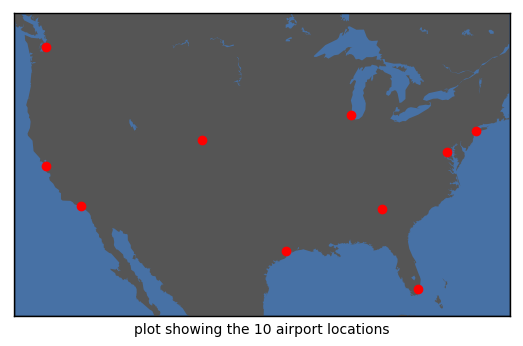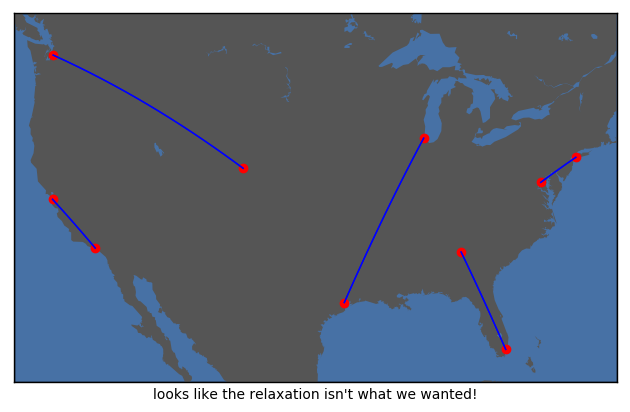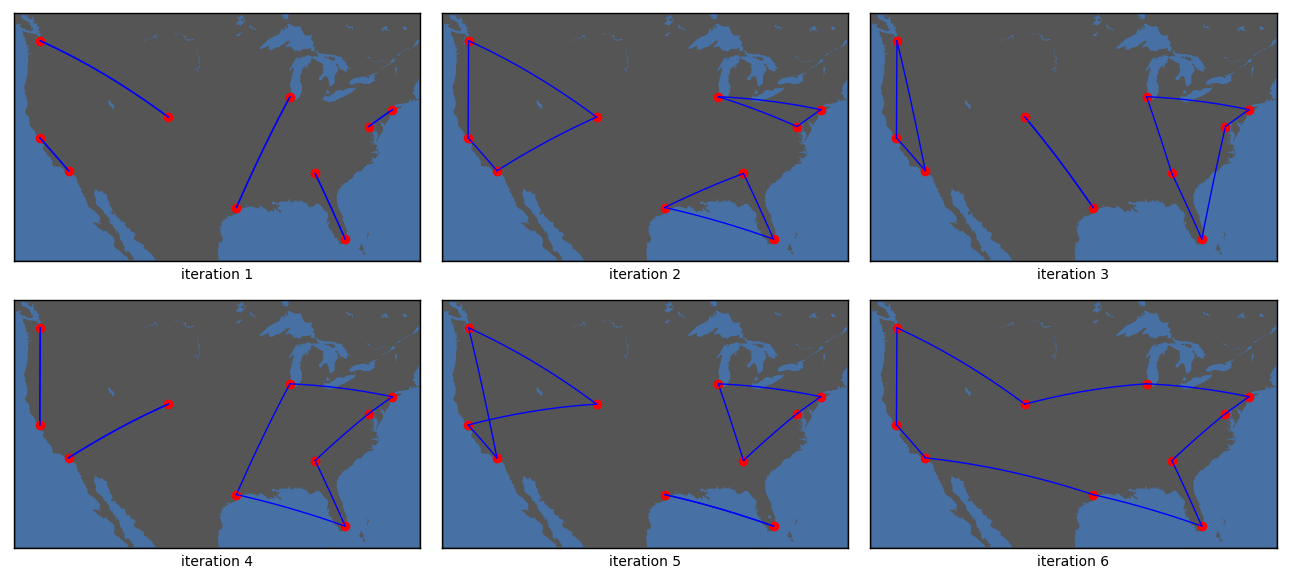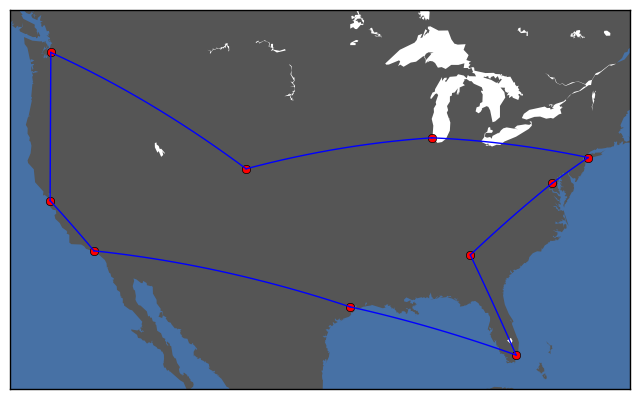# Traveling salesman problem¶

Find the closed tour that visits all ten cities and has the minimum total length. Distances between pairs of cities are given in the table below:

ATL ORD DEN IAH LAX MIA JFK SFO SEA DCA
ATL 0 587 1212 701 1936 604 748 2139 2182 543
ORD 587 0 920 940 1745 1188 713 1858 1737 597
DEN 1212 920 0 879 831 1726 1631 949 1021 1494
IAH 701 940 879 0 1379 968 1420 1645 1891 1220
LAX 1936 1745 831 1379 0 2339 2451 347 959 2300
MIA 604 1188 1726 968 2339 0 1092 2594 2734 923
JFK 748 713 1631 1420 2451 1092 0 2571 2408 205
SFO 2139 1858 949 1645 347 2594 2571 0 678 2442
SEA 2182 1737 1021 1891 959 2734 2408 678 0 2329
DCA 543 597 1494 1220 2300 923 205 2442 2329 0
In :
# Define the problem data (cities and costs)
# Run this, then run the final block of this notebook to load the helper functions before continuing
using JuMP, NamedArrays, Cbc

cities = [:ATL, :ORD, :DEN, :IAH, :LAX, :MIA, :JFK, :SFO, :SEA, :DCA]

distances = [     0   587  1212   701  1936   604   748  2139  2182   543
587     0   920   940  1745  1188   713  1858  1737   597
1212   920     0   879   831  1726  1631   949  1021  1494
701   940   879     0  1379   968  1420  1645  1891  1220
1936  1745   831  1379     0  2339  2451   347   959  2300
604  1188  1726   968  2339     0  1092  2594  2734   923
748   713  1631  1420  2451  1092     0  2571  2408   205
2139  1858   949  1645   347  2594  2571     0   678  2442
2182  1737  1021  1891   959  2734  2408   678     0  2329
543   597  1494  1220  2300   923   205  2442  2329     0  ]

c = NamedArray(distances,(cities,cities))
N = size(distances,1);

In :
# Run the final block of this notebook to load helper functions before continuing!
mapSolution(0)
xlabel("plot showing the 10 airport locations");## Solve TSP using min-cost flow relaxation¶

In :
# solve the simplest version (min-cost flow version; it's an assignment problem)

m = Model(solver = CbcSolver())
@variable(m, 0 <= x[cities,cities] <= 1)                                 # LP relaxation
@constraint(m, c1[j in cities], sum( x[i,j] for i in cities ) == 1)    # exacly one edge out of each node
@constraint(m, c2[i in cities], sum( x[i,j] for j in cities ) == 1)    # exactly one edge into each node
@constraint(m, c3[i in cities], x[i,i] == 0 )                       # no self-loops
@objective(m, Min, sum( x[i,j]*c[i,j] for i in cities, j in cities ))  # minimize total cost
solve(m)

# pretty print the solution
xx = getvalue(x)
sol = NamedArray(zeros(Int,N,N),(cities,cities))
for i in cities
for j in cities
sol[i,j] = Int(xx[i,j])
end
end
println(sol)
sleep(1)
display(getAllSubtours(sol))
mapSolution(sol)
xlabel("looks like the relaxation isn't what we wanted!")
tight_layout()
#savefig("tsp_sol_LP.pdf")
;

10×10 Named Array{Int64,2}
A ╲ B │ :ATL  :ORD  :DEN  :IAH  :LAX  :MIA  :JFK  :SFO  :SEA  :DCA
──────┼───────────────────────────────────────────────────────────
:ATL  │    0     0     0     0     0     1     0     0     0     0
:ORD  │    0     0     0     1     0     0     0     0     0     0
:DEN  │    0     0     0     0     0     0     0     0     1     0
:IAH  │    0     1     0     0     0     0     0     0     0     0
:LAX  │    0     0     0     0     0     0     0     1     0     0
:MIA  │    1     0     0     0     0     0     0     0     0     0
:JFK  │    0     0     0     0     0     0     0     0     0     1
:SFO  │    0     0     0     0     1     0     0     0     0     0
:SEA  │    0     0     1     0     0     0     0     0     0     0
:DCA  │    0     0     0     0     0     0     1     0     0     0

5-element Array{Any,1}:
Symbol[:ATL, :MIA, :ATL]
Symbol[:ORD, :IAH, :ORD]
Symbol[:DEN, :SEA, :DEN]
Symbol[:LAX, :SFO, :LAX]
Symbol[:JFK, :DCA, :JFK]## Solve TSP using adaptive subtour elimination¶

In :
m = Model(solver = CbcSolver())
@variable(m, x[cities,cities], Bin)                                       # must formulate as IP this time
@constraint(m, c1[j in cities], sum( x[i,j] for i in cities ) == 1)     # one out-edge
@constraint(m, c2[i in cities], sum( x[i,j] for j in cities ) == 1)     # one in-edge
@constraint(m, c3[i in cities], x[i,i] == 0 )                        # no self-loops
@objective(m, Min, sum( x[i,j]*c[i,j] for i in cities, j in cities ))   # minimize total cost

sols = []

for iters = 1:30
solve(m)
println("Tour length: ", getobjectivevalue(m))
xx = getvalue(x)
push!(sols,xx)
subtours = getAllSubtours(xx)  # get all the subtours
display(subtours)
sleep(1)
len = length(subtours)
if len == 1                    # solution is just a single tour!
println("SOLVED!")
break
else
for subtour in subtours
L = length(subtour)
@constraint(m, sum( x[subtour[k+1],subtour[k]] for k = 1:L-1 ) <= L-2)
@constraint(m, sum( x[subtour[k],subtour[k+1]] for k = 1:L-1 ) <= L-2)
end
end
end

5-element Array{Any,1}:
Symbol[:ATL, :MIA, :ATL]
Symbol[:ORD, :IAH, :ORD]
Symbol[:DEN, :SEA, :DEN]
Symbol[:LAX, :SFO, :LAX]
Symbol[:JFK, :DCA, :JFK]
Tour length: 6234.0

WARNING: Solver does not appear to support adding constraints to an existing model. JuMP's internal model will be discarded.

3-element Array{Any,1}:
Symbol[:ATL, :MIA, :IAH, :ATL]
Symbol[:ORD, :JFK, :DCA, :ORD]
Symbol[:DEN, :SEA, :SFO, :LAX, :DEN]
Tour length: 6665.0

3-element Array{Any,1}:
Symbol[:ATL, :MIA, :DCA, :JFK, :ORD, :ATL]
Symbol[:DEN, :IAH, :DEN]
Symbol[:LAX, :SEA, :SFO, :LAX]            
Tour length: 6774.0

3-element Array{Any,1}:
Symbol[:ATL, :DCA, :JFK, :ORD, :IAH, :MIA, :ATL]
Symbol[:DEN, :LAX, :DEN]
Symbol[:SFO, :SEA, :SFO]                        
Tour length: 6991.0

3-element Array{Any,1}:
Symbol[:ATL, :DCA, :JFK, :ORD, :ATL]
Symbol[:DEN, :SFO, :LAX, :SEA, :DEN]
Symbol[:IAH, :MIA, :IAH]            
Tour length: 7260.0

1-element Array{Any,1}:
Symbol[:ATL, :DCA, :JFK, :ORD, :DEN, :SEA, :SFO, :LAX, :IAH, :MIA, :ATL]
Tour length: 7378.0
SOLVED!

In :
# plot the iterations as we eliminate subtours
figure(figsize=(13,6))
for i = 1:6
subplot(2,3,i)
mapSolution(sols[i])
xlabel(string("iteration ",i))
end
tight_layout()
#savefig("tsp_sol_elim.pdf")## Miller-Tucker-Zemlin formulation¶

In :
m = Model(solver = CbcSolver())
@variable(m, x[cities,cities], Bin)                                      # must formulate as IP this time
@constraint(m, c1[j in cities], sum( x[i,j] for i in cities ) == 1)      # one out-edge
@constraint(m, c2[i in cities], sum( x[i,j] for j in cities ) == 1)      # one in-edge
@constraint(m, c3[i in cities], x[i,i] == 0 )                            # no self-loops
@objective(m, Min, sum( x[i,j]*c[i,j] for i in cities, j in cities ))   # minimize total cost

# MTZ variables and constraints
@variable(m, u[cities])
@constraint(m, c4[i in cities, j in cities[2:end]], u[i] - u[j] + N*x[i,j] <= N-1 )

solve(m)
xx = getvalue(x)
subtours = getAllSubtours(xx)  # get cycle containing Atlanta
display(subtours)
println("Tour length: ", getobjectivevalue(m))

1-element Array{Any,1}:
Symbol[:ATL, :MIA, :IAH, :LAX, :SFO, :SEA, :DEN, :ORD, :JFK, :DCA, :ATL]
Tour length: 7378.0

In :
mapSolution(xx)## Helper functions¶

### NOTE¶

I got this to work by following these steps (Windows machine)

ENV["PYTHON"]=""        # use Julia-specific python distribution
Pkg.build("PyCall")     # rebuild the PyCall interface
using Conda
Conda.add("mpl_toolkits.basemap")
Conda.update()

You can run Conda.list() to ensure that you have the correct version of basemap installed. It should be version 1.1.0. The reason is that basemap depends on matplotlib. The latter was updated recently and they deprecated the function get_axis_bgcolor(), which breaks basemap. The latest version 1.1.0 of basemap fixes the issue.

In my case, the steps above only installed 1.0.7. After some looking online, I found out how to get the latest version. First, open a command prompt and navigate to the directory containing the conda script. In my case, it was:

C:\Users\Laurent\AppData\Local\JuliaPro-0.6.2.1\pkgs-0.6.2.1\v0.6\Conda\deps\usr\Scripts

Then, run the following two commands (each will take a bit of time)

conda install -c conda-forge basemap
conda install -c conda-forge basemap-data-hires

After this is done, you can return to Julia and check using Conda.list() that basemap 1.1.0 is installed. Finally, restart the kernel and everything should work.

In :
# HELPER FUNCTION: returns the cycle containing the city START.
function getSubtour(x,start)
subtour = [start]
while true
j = subtour[end]
for k in cities
if x[k,j] == 1
push!(subtour,k)
break
end
end
if subtour[end] == start
break
end
end
return subtour
end

# HELPER FUNCTION: returns a list of all cycles
function getAllSubtours(x)
nodesRemaining = cities
subtours = []
while length(nodesRemaining) > 0
subtour = getSubtour(x,nodesRemaining)
push!(subtours, subtour)
nodesRemaining = setdiff(nodesRemaining,subtour)
end
return subtours
end

# HELPER FUNCTION FOR MAPPING AIRPORTS

data = [ 33.636700  -84.427863
41.977320  -87.908005
39.861667 -104.673166
29.645417  -95.278888
33.942437 -118.408121
25.795361  -80.290110
40.639926  -73.778694
37.618806 -122.375416
47.449889 -122.311777
38.851444  -77.037721 ]
lat = Dict(zip(cities,data[:,1]))
lon = Dict(zip(cities,data[:,2]))

using PyPlot, PyCall
@pyimport mpl_toolkits.basemap as basemap

function mapSolution(x=0)
m=basemap.Basemap(projection="merc", resolution="l",llcrnrlat=23,llcrnrlon=-126,urcrnrlat=50,urcrnrlon=-70)
m[:drawmapboundary](fill_color="#4771a5")
m[:fillcontinents](color="#555555")

# plot airports
for i in cities
m[:plot](lon[i], lat[i], "ro" ,latlon=true)
end

# plot tours
if x==0
return
else
tours = getAllSubtours(x)
for t in tours
L = length(t)-1
for i in 1:L
m[:drawgreatcircle](lon[t[i]],lat[t[i]],lon[t[i+1]],lat[t[i+1]],linewidth=1,color="b")
end
end
end
end
;

In [ ]: